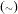MODEL Statement

MODEL dependent-variabledistribution ;

The MODEL statement is the mechanism for specifying the conditional distribution of the data given the random effects. You must specify a single dependent variable from the input data set, a tilde, and then a distribution with its parameters. Valid distributions are as follows.

• normal(m,v) specifies a normal (Gaussian) distribution with meanand variance.

• binary(p) specifies a binary (Bernoulli) distribution with probability.

• binomial(n,p) specifies a binomial distribution with countand probability.

• gamma(a,b) specifies a gamma distribution with shapeand scale.

• negbin(n,p) specifies a negative binomial distribution with countand probability.

• poisson(m) specifies a Poisson distribution with mean.

• general(ll) specifies a general log likelihood function that you construct using SAS programming statements.

The MODEL statement must follow any SAS programming statements you specify for computing parameters of the preceding distributions. See the section Built-in Log-Likelihood Functions for expressions of the built-in conditional log-likelihood functions.